# Regulate a boost converter step by step

#### Zouglou LeMagicien

Joined Feb 12, 2019
33
Hello,

I want to regulate my boost step by step, for this I need to control the voltage that the inductor can induce to the capacitor. For example, if I want a step of 5V, I want my inductor to deliver a voltage of 5V (+ the supply) to the capacitor.
So, I tried a simulation (attach file below) with that kind of parameters :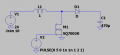There is only 1 pulse that lasts 1 second.

During the pulse, I have a current of ~1.66A, so if I made the equation of the voltage:
V = L di/dt = 1* 1.66/1 = 1.66V
But when the pulse turn off and the inductor start discharging (charging the capacitor at the same time), I got a very high voltage across the capacitor: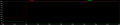The voltage across the capacitor goes up to 100V which is not what I want. I wanted here to have a step-up of 1.66V per pulse...

Does anyone know why it doesn't work ?

Chris

#### Attachments

• 886 bytes Views: 3
Last edited:

#### hrs

Joined Jun 13, 2014
329
The switching time dt in your calculation is 1 second. Looking at the graph it's clearly much shorter so you will get a higher voltage.

•Zouglou LeMagicien

#### Zouglou LeMagicien

Joined Feb 12, 2019
33
Is there a relationship bewteen the capacitor and the inductor to say that I want only step up by 5v ?

#### MrAl

Joined Jun 17, 2014
8,353
Hello,

I want to regulate my boost step by step, for this I need to control the voltage that the inductor can induce to the capacitor. For example, if I want a step of 5V, I want my inductor to deliver a voltage of 5V (+ the supply) to the capacitor.
So, I tried a simulation (attach file below) with that kind of parameters :
View attachment 207224
There is only 1 pulse that lasts 1 second.

During the pulse, I have a current of ~1.66A, so if I made the equation of the voltage:
V = L di/dt = 1* 1.66/1 = 1.66V
But when the pulse turn off and the inductor start discharging (charging the capacitor at the same time), I got a very high voltage across the capacitor:
View attachment 207227

The voltage across the capacitor goes up to 100V which is not what I want. I wanted here to have a step-up of 1.66V per pulse...

Does anyone know why it doesn't work ?

Chris

You should really include the load resistance here because when the mosfet is on the capacitor discharges into the load resistance as the inductor charges up again. Thus the output voltage ramps up and down slightly with each cycle.
As the average output voltage changes, the increase per pulse will decrease so if you really want a perfect step of some voltage like 5v then you will have to also alter the pulse width (turn on time of the mosfet).
If this sounds ok we can work out the equations.
If you really want to "regulate" this step rather than pre-calculate it then it gets more complicated because then we have to implement some system of dynamic measurement. It should be possible though.

#### ronsimpson

Joined Oct 7, 2019
1,312
Changes" L1=1mH, C1 changed ESR to 0.1 ohms, On time =1mS, (edited to fix typo)
Red trace is the current in L1. Starts at 0 and goes to 2.4A in 1mS. (edited to fix typo)
With M1 off the current slops back to 0 by 1.5mS.
This current pushes up the voltage on C1 from less than 24V to 27V.
If there was a second pulse it would also push C1 up about that much more.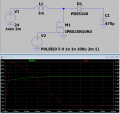#### Attachments

• 957 bytes Views: 2
Last edited:
•Zouglou LeMagicien

#### MrAl

Joined Jun 17, 2014
8,353
Changes" L1=1mH, C1 changed ESR to 0.1 ohms, On time =1nS,
Red trace is the current in L1. Starts at 0 and goes to 2.4A in 1nS.
With M1 off the current slops back to 0 by 1.5mS.
This current pushes up the voltage on C1 from less than 24V to 27V.
If there was a second pulse it would also push C1 up about that much more.
View attachment 207243
Hi,

A 1ns pulse, seriously?What is the rise time of the mosfet, 1ps?#### ronsimpson

Joined Oct 7, 2019
1,312
A 1ns pulse, seriously?
Sorry, on the graph you can see 1mS. Typo - seriously.

Last edited:

#### Alec_t

Joined Sep 17, 2013
12,148
Is there a relationship bewteen the capacitor and the inductor to say that I want only step up by 5v ?
The voltage generated when the inductor current is reduced is -L di/dt. So to limit that voltage to 5V you would have to provide a controlled current reduction. It is the trailing edge of your 1 sec pulse which is important here; not the pulse width. The pulse width does however affect the amount of energy 0.5 L i^2 J stored in the inductor for transfer to the cap.
The energy stored in the cap is 0.5 C V^2 J, so to increase the cap voltage by 5V would require an increase in energy of 0.5 C ((Vo+5)^2 - Vo^2) J, where Vo is the initial voltage.

Last edited:
•Zouglou LeMagicien

#### MrAl

Joined Jun 17, 2014
8,353
Sorry on the graph you can see 1mS. Typo - seriously.
Oh ok ha ha•ronsimpson

#### Zouglou LeMagicien

Joined Feb 12, 2019
33
You should really include the load resistance here because when the mosfet is on the capacitor discharges into the load resistance as the inductor charges up again. Thus the output voltage ramps up and down slightly with each cycle.
As the average output voltage changes, the increase per pulse will decrease so if you really want a perfect step of some voltage like 5v then you will have to also alter the pulse width (turn on time of the mosfet).
If this sounds ok we can work out the equations.
If you really want to "regulate" this step rather than pre-calculate it then it gets more complicated because then we have to implement some system of dynamic measurement. It should be possible though.
Currently the output is only another capacitor who will be used for a double pulse test.
And I don't want a perfect 5V step for example that will just be arround a value#### Zouglou LeMagicien

Joined Feb 12, 2019
33
The voltage generated when the inductor current is reduced is -L di/dt. So to limit that voltage to 5V you would have to provide a controlled current reduction. It is the trailing edge of your 1 sec pulse which is important here; not the pulse width. The pulse width does however affect the amount of energy 0.5 L i^2 J stored in the inductor for transfer to the cap.
The energy stored in the cap is 0.5 C V^2 J, so to increase the cap voltage by 5V would require an increase in energy of 0.5 C ((Vo+5)^2 - Vo^2) J, where Vo is the initial voltage.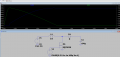Here I have a step up of 1.6V for a current of 1.6A
If I make the calcul via the energy I got:
Energy to increase : E = 0.5 *470µ ((24+1.6)^2 - 24^2) = 18.65mJ
Energy in the inductor = Energy to increase = 18.65mJ = Li²/2
Current that need the inductor = I = sqrt(18.65m*2/10^-3) = 6.11A
The current that I get isn't 1.6A that I use on my simulation...

Where do I get wrong ?

#### Alec_t

Joined Sep 17, 2013
12,148
The current value used to calculate the energy stored in the inductor is the peak inductor current.

Last edited:

#### Zouglou LeMagicien

Joined Feb 12, 2019
33
The current value used to calculate the energy stored in the inductor is the peak inductor current.
Yes but the peak here on my screenshot is currently 1.6A no ?

Last edited:

#### ronsimpson

Joined Oct 7, 2019
1,312
(24+1.6)^2 - 24^2
The energy going into the cap is 0.5 x 470u x 1.6^2. The voltage change is 1.6. Do not count the 24V, that energy was there before you started.

Energy in the inductor: If it was a square wave (starts at 1.6A and ends at 1.6A) then I^2. But I think you need to take into account the current starts at 1.6A and ramps down to 0A so it is 1/2 of what you think.

Have not had my coffee yet so may be really wrong! [insert happy face here but its too early]
-----edited------
I think the capacitor comment is right but the inductor comment is wrong.

Last edited:
•Zouglou LeMagicien

#### Zouglou LeMagicien

Joined Feb 12, 2019
33
The energy going into the cap is 0.5 x 470u x 1.6^2. The voltage change is 1.6. Do not count the 24V, that energy was there before you started.

Energy in the inductor: If it was a square wave (starts at 1.6A and ends at 1.6A) then I^2. But I think you need to take into account the current starts at 1.6A and ramps down to 0A so it is 1/2 of what you think.

Have not had my coffee yet so may be really wrong! [insert happy face here but its too early]
-----edited------
I think the capacitor comment is right but the inductor comment is wrong.
I check it on Ltspice by the simulation of the energy: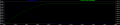The comment of the capacitor that you made seems wrong...
By checking the simulation we have at the beginning ~136mJ and at the end ~154mJ so an increase of ~18mJ
When we make 0.5 x 470u x 1.6^2, we got an increase of 0.6mJ
But when we make the difference of the energy at 25.6V and the energy at 24V, we got an increase of 18.65mJ which is what we have at our simulation

#### MrAl

Joined Jun 17, 2014
8,353
One of the things that you have to think about is how the diode and mosfet itself affect the output.
With just a power source, ideal switch, inductor and capacitor, the circuit is ideal, but once you add the diode and mosfet it becomes non ideal and thus there will be losses and those losses will depend highly on the resistive energy eaters.
If you want to see the ideal behavior you probably have to replace the mosfet and the diode with ideal switches creating a synchronous converter.

The actual real circuit equations are a little complicated i am not sure they would do you any good or not.

•Zouglou LeMagicien

#### MrAl

Joined Jun 17, 2014
8,353
Hello again,

Oh one of the things i forgot to mention was that the energy into the inductor is (1/2)*i^2*L and that is dumped into the capacitor (1/2)*v^2*C but the equation:
(1/2)*i^2*L=(1/2)*v^2*C
does not hold because although the energy during the mosfet 'on' time is the left hand side there, the energy dumped into the cap is not the right hand side. That is because as the energy from the inductor is being dumped into the cap there is more energy being supplied to the system from the input voltage source. So we really have this:
(1/2)*i^2*L+W=(1/2)*v^2*C
where W is that extra energy and this means that the voltage across the cap after the very first cycle is going to be higher than without that extra energy.
So next we'd have to calculate the extra energy and add that to the total energy that gets dumped into the cap and then calculate the voltage.

What seems to work is the energy equation looks like it holds AFTER the first cycle or so.
But the energy equation changes to:
(1/2)*i^2*L=(vb-24)^2-(va-24)^2*C/2
and from that we can calculate vb, or so it looks as such.
I'll have to check this over with the exact equations to make sure this works all the time though.
In any case, i think you have to use the exact equations to get the voltage for the first cycle because the inductor current waveshape is partly sinusoidal, enough so that the regular energy equation doesnt help.

Last edited:
•Zouglou LeMagicien

#### MrAl

Joined Jun 17, 2014
8,353
Hello again,

I thought i would post some actual numbers so that you can compare and see if you get the same results.
These results agree very closely with a simulation using a diode that has about 8mv forward voltage drop (that's 0.008v at 24 amps). The diode was made special so that the results of the simulation agree very well with the theoretical calculations. I only did three points so far but that should be good enough to get started.
The voltages are the voltage after each cycle that the inductor has discharged, and the operation is assumed to be only discontinuous throughout.

Code:
66.4444568217526 volts
79.0187588899208 volts
89.2119294660176 volts
Next we might try to calculate the pulse width required for each step. or we might just provide feedback that actually regulates the height of each step.
We could also investigate if the correlation between the exact solutions above and the energy equations come out close enough after some cycles.

I'll also post the exact equations if anyone else wants to try this. It is interesting to do at least once in your lifetimeIn the following, each set of expressions is for the current through the inductor and the voltage of the output.
The arrangement is
[iL,vC]
so there are two expressions for each mosfet switch state, one for current and one for voltage.
Starting with the first cycle we calculate the On values, then insert them into the Off expressions for i and v respectively, then calculate the voltage and current at the end of the first complete cycle.
We then move to the second cycle, starting with the On expressions and using the current and voltage from the previous Off expressions, then calculate the new On values. Then we do the Off expressions again using the vlaues of current and voltage from the previous On expressions, and we have the voltage at the end of the second cycle.
We repeat this process for as many cycles as we wish to calculate.
It is very note worthy to mention that at the beginning of each Off partial cycle we always start with i=24 amps and at the end of each Off cycle we always end up with 0 amps. This is due to the discontinuous operation and of course the input voltage, inductor value, and On time.
The only caveat here is that in order to get the time for the end of each Off cycle, we must solve for the time value before we can calculate the voltage at the end of that cycle. To do this, we just equate the current to zero and solve for 't' the time variable, then we can use that in the Off voltage expression to get the actual ending voltage.

Just as a reminder, there are two expressions for On(t) and two for Off(t). I posted these in 'code' tags to try to make them more clear, and you should be able to copy and paste them into some other math program and follow the procedure above to calculate the output voltage for as many cycles as you wish.

Code:
On(t):[(t*E)/L+i,v];
Off(t):[(sqrt(C)*E*sin(t/(sqrt(C)*sqrt(L))))/sqrt(L)-(v*sqrt(C)*sin(t/(sqrt(C)*sqrt(L))))/sqrt(L)-(vd*cos(t/(sqrt(C)*sqrt(L))))/L+i*cos(t/(sqrt(C)*sqrt(L))),(i*sin(t/(sqrt(C)*sqrt(L)))*sqrt(L))/sqrt(C)-(vd*sin(t/(sqrt(C)*sqrt(L))))/(sqrt(C)*sqrt(L))-E*cos(t/(sqrt(C)*sqrt(L)))+v*cos(t/(sqrt(C)*sqrt(L)))+E];

Last edited:
•Zouglou LeMagicien

#### ronsimpson

Joined Oct 7, 2019
1,312